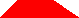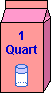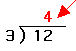EnchantedLearning.com is a user-supported site.
As a bonus, site members have access to a banner-ad-free version of the site, with print-friendly pages.

 +, - EnchantedLearning.comMath Dictionary x, ÷
 A B C D E F G H I J K L M N O P Q R S T U V W X Y ZQ is for ...Q.E.D.Q.E.D. is an abbreviation of the Latin phrase "quod erat demonstrandum" (meaning "that which was to be demonstrated"). Q.E.D. can be written at the end of a mathematical proof to show that the proof is complete.quadrantA quadrant is a quarter of a plane. The x-axis and y-axis divide the x-y plane into four quadrants. The axes themselves are not part of the quadrants.quadratic equationA quadratic equation is an equation that has a second-degree term and no higher terms. A second-degree term is a variable raised to the second power, like x2, or the product of exactly two variables, like x and y. When you graph a quadratic equation in one variable, like y = ax2 + bx + c, you get a parabola, and the solutions to the quadratic equation represent the points where the parabola crosses the x-axis.quadratic formulaThe quadratic formula is a formula that gives you a solution to the quadratic equation ax2 + bx + c = 0. The quadratic formula is obtained by solving the general quadratic equation.quadrilateralA quadrilateral is a four-sided figure. The square, rectangle, rhombus, trapezoid, kite, and parallelogram are quadrilaterals.quartA quart is one-fourth of a gallon. Four quarts make one gallon.quarterA quarter is a coin that is worth 25 cents. Four quarters make one dollar. quartileA quartile for a data set is one of three data points that divide the set of data into four parts, each containing a quarter of the data. The first point marks the lower quartile boundary at the 25th percentile. The second marks the middle quartile (or midpoint of the data set), the median or the 50th percentile. The last marks the upper quartile (or 75th percentile) of a frequency distribution.quotientThe quotient is the answer in long division. The dividend divided by the divisor is the quotient (plus a remainder).

 +, - EnchantedLearning.comMath Dictionary x, ÷
 A B C D E F G H I J K L M N O P Q R S T U V W X Y Z
 Number Line Fractions Decimals Graphs Measurement Rounding

Click on an underlined word for more information on that subject. If the math term you are looking for is not in the dictionary, please e-mail us.

Enchanted Learning®
Over 35,000 Web Pages
Sample Pages for Prospective Subscribers, or click below

 Overview of Site What's New Enchanted Learning Home Monthly Activity Calendar Books to Print Site Index K-3 Crafts K-3 Themes Little ExplorersPicture dictionary PreK/K Activities Rebus Rhymes Stories Writing Cloze Activities Essay Topics Newspaper Writing Activities Parts of Speech Fiction The Test of Time Biology Animal Printouts Biology Label Printouts Biomes Birds Butterflies Dinosaurs Food Chain Human Anatomy Mammals Plants Rainforests Sharks Whales Physical Sciences: K-12 Astronomy The Earth Geology Hurricanes Landforms Oceans Tsunami Volcano Languages Dutch French German Italian Japanese (Romaji) Portuguese Spanish Swedish Geography/History Explorers Flags Geography Inventors US History Other Topics Art and Artists Calendars College Finder Crafts Graphic Organizers Label Me! Printouts Math Music Word Wheels

## Enchanted Learning Search

 Search the Enchanted Learning website for: